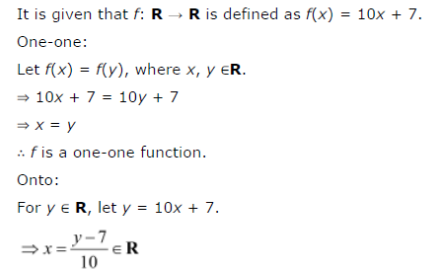# Let f : R → R be defined as f(x) = 10x + 7. Find the function such that gof = fog = IR

Let f : R → R be defined as f(x) = 10x + 7. Find the function such that gof = fog = IR.